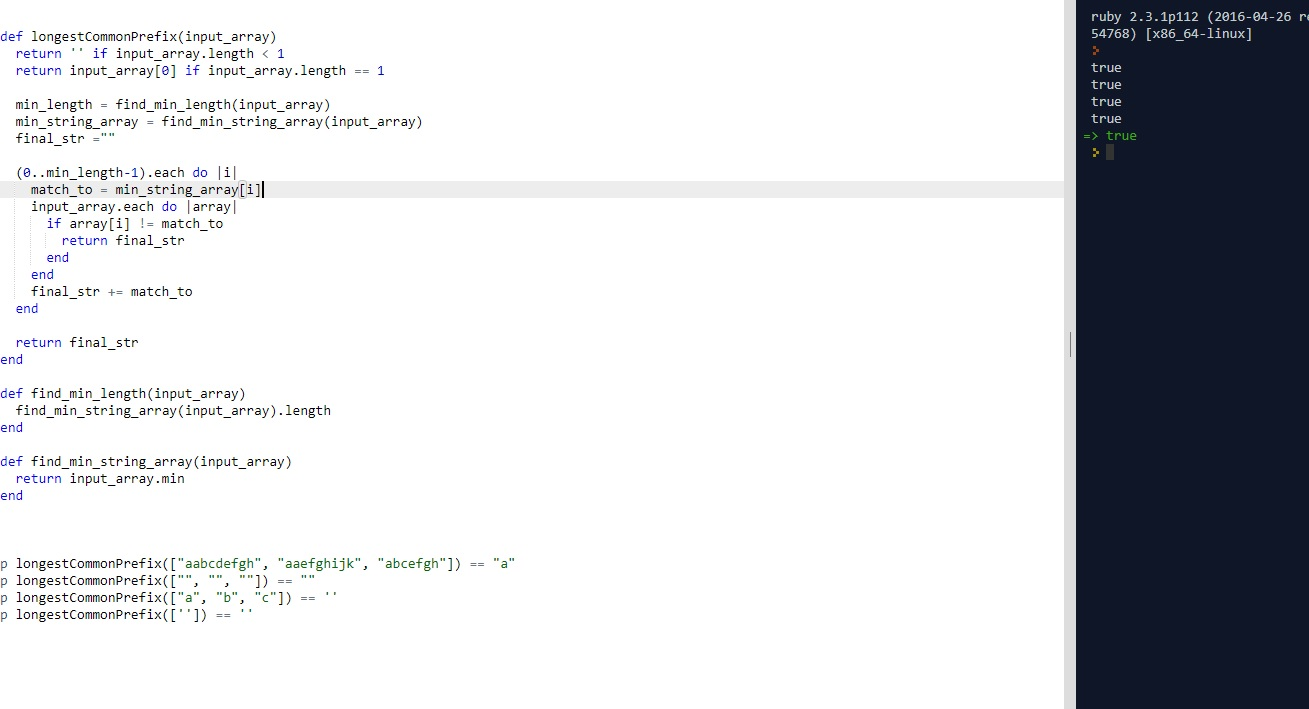# CLEAN CODE: LONGEST COMMON PREFIX

By: Saurav

2017-11-08 21:05:00 UTC

Write a function to find the longest common prefix string amongst an array of strings.

Longest common prefix for a pair of strings S1 and S2 is the longest string S which is the prefix of both S1 and S2.

As an example, longest common prefix of "abcdefgh" and "abcefgh" is "abc".

Given the array of strings, you need to find the longest S which is the prefix of ALL the strings in the array.

Example:

Given the array as:

[

"abcdefgh",

"aefghijk",

"abcefgh"
]
The answer would be “a”.

InterviewBit

As always, lets start with writing some tests first:

```p find_longest_common_prefix(["aabcdefgh", "aaefghijk", "abcefgh"]) == "a"
p find_longest_common_prefix(["", "", ""]) == ""
p find_longest_common_prefix(["a", "b", "c"]) == ''
p find_longest_common_prefix(['']) == ''
p find_longest_common_prefix([' a', 'b ']) == ''```

Attempt 1
1. My idea is to choose the minimum element from the array
2. Iterate through its element and compare that element with each element of the input array
3. If its a match with all of them, append the common elements stirng
4. If not, return the common element string built so far

Note: In ruby , array.min will return minimum element with respect to lexicographic order so array.min won't work here.
According to This Post better use min_by(&:length)
which is equivalent to .min_by {|x| x.length }

A reminder: Post
In a method argument list, the & operator takes its operand, converts it to a Proc object if it isn't already (by calling to_proc on it) and passes
it to the method as if a block had been used.
my_proc = Proc.new { puts "foo" }
my_method_call(&my_proc) # is identical to:
my_method_call { puts "foo" }

```def longestCommonPrefix(input_array)
return '' if input_array.length < 1
return input_array if input_array.length == 1

min_length = find_min_length(input_array)
min_string_array = find_min_string_array(input_array)
final_str =""

(0..min_length-1).each do |i|
match_to = min_string_array[i]
input_array.each do |array|
if array[i] != match_to
return final_str
end
end
final_str += match_to
end

return final_str
end

def find_min_length(input_array)
find_min_string_array(input_array).length
end

def find_min_string_array(input_array)
return input_array.min_by(&:length)
end

p longestCommonPrefix(["aabcdefgh", "aaefghijk", "abcefgh"]) == "a"
p longestCommonPrefix(["", "", ""]) == ""
p longestCommonPrefix(["a", "b", "c"]) == ''
p longestCommonPrefix(['']) == ''```As we see, all our tests pass. But I don't really like this code! Lets make it a bit cleaner:

Attempt 2:

```def find_longest_common_prefix(input_array)
return input_array.join if input_array.length < 2
min_length, min_string_array, final_str = find_min_length(input_array), find_min_string_array(input_array), ""
(0..min_length-1).each do |index|
final_str = match_with_each_string(input_array- [min_string_array] , final_str, min_string_array[index], index)
end

return final_str
end

def find_min_length(input_array)
find_min_string_array(input_array).length
end

def find_min_string_array(input_array)
return input_array.min_by(&:length)
end

def match_with_each_string(input_array,final_str, match_to,index)
input_array.each do |array|
return final_str if array[index] != match_to
end
final_str = append_final_string(final_str, match_to)
return final_str
end

def append_final_string(final_str, match_to)
final_str += match_to
end ```

Looks a bit cleaner, each function doing just one thing and following single responsibility principle and we are removing extra iterations and comparisons as well.

Let me know if you have a better more cleaner way and I will add your suggestions as well :)

Owned & Maintained by Saurav Prakash

If you like what you see, you can help me cover server costs or buy me a cup of coffee though donation :)